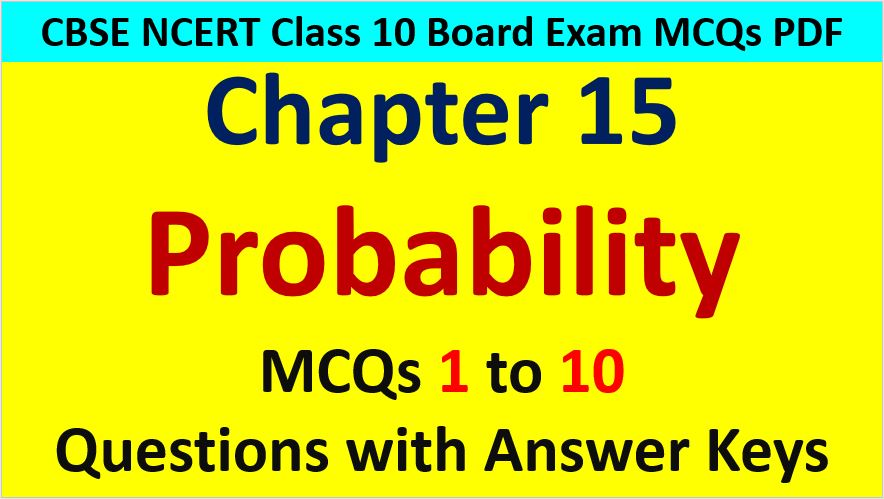Friday, October 22, 2021
Home > CBSE Class 10 > MCQ Questions for Class 10 Maths Chapter 15 Probability with Answer Keys

# MCQ Questions for Class 10 Maths Chapter 15 Probability with Answer KeysHi students, Welcome to AMBiPi (Amans Maths Blogs). In this article, you will get MCQ Questions for Class 10 Maths Chapter 15 Probability with Answer Keys PDF. You can download this PDF and save it in your mobile device or laptop etc.

MCQ Questions for Class 10 Maths Chapter 15 Probability Question No 1:

The probability of getting exactly one head in tossing a pair of coins is

Option A : 0

Option B : 1

Option C : 1/3

Option D : 1/2

Option D : 1/2

MCQ Questions for Class 10 Maths Chapter 15 Probability Question No 2:

Which of the the following can be the probability of an event?

Option A : – 0.04

Option B : 1.004

Option C : 18/23

Option D : 8/7

Option C : 18/23

MCQ Questions for Class 10 Maths Chapter 15 Probability Question No 3:

The probability that a number selected at random from the numbers 1, 2, 3, ……, 15 is a multiple of 4 is

Option A : 4/15

Option B : 2/15

Option C : 1/15

Option D : 1/5

Option D : 1/5

MCQ Questions for Class 10 Maths Chapter 15 Probability Question No 4:

The probability of getting a spade card from a well shuffled deck of 52 cards is

Option A : 1/13

Option B : 1/4

Option C : 12/13

Option D : 3/4

Option D : 1/4

MCQ Questions for Class 10 Maths Chapter 15 Probability Question No 5:

A card is selected at random from a well shuffled deck of 52 playing cards. The probability of its being a face card is

Option A : 3/13

Option B : 4/13

Option C : 6/13

Option D : 9/13

Option A : 3/13

MCQ Questions for Class 10 Maths Chapter 15 Probability Question No 6:

Two coins are tossed simultaneously. The probability of getting at most one head is

Option A : 1/4

Option B : 1/2

Option C : 2/3

Option D : 3/4

Option D : 3/4

MCQ Questions for Class 10 Maths Chapter 15 Probability Question No 7:

The probability of getting less than 3 in a single throw of a die is

Option A : 1/3

Option B : 1/2

Option C : 1/4

Option D : 2/3

Option A : 1/3

MCQ Questions for Class 10 Maths Chapter 15 Probability Question No 8:

A bag contains 3 red balls, 5 white balls and 7 black balls. What is the probability that a ball drawn from the bag at random will be neither red nor black?

Option A : 1/5

Option B : 1/3

Option C : 7/15

Option D : 8/15

Option B : 1/3

MCQ Questions for Class 10 Maths Chapter 15 Probability Question No 9:

An event is very unlikely to happen. Its probability is closest to

Option A : 0.0001

Option B : 0.001

Option C : 0.01

Option D : 0.1

Option A : 0.0001

MCQ Questions for Class 10 Maths Chapter 15 Probability Question No 10:

The total number of events of throwing 10 coins simultaneously is

Option A : 1024

Option B : 512

Option C : 256

Option D : 128